Next: Conclusions Up: Theoretical models Previous: Second-order perturbation results

In the previous sections, we have considered models having either degenerate (Sec. 3.2) or arbitrarily fixed (Secs. 3.3 and 3.4) single-particle spectra. However, in real systems, the single-particle energies do depend on the numbers of particles, and the energy-spacing filters (7) extract from masses the single-particle energies which include the particle-number dependence, i.e, at every N, the distance to the next available single-particle level is obtained.

In order to account for such effects, in this section we analyze the results of the exact diagonalization of the pairing-plus-quadrupole (PPQ) Hamiltonian,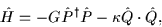(64)

in a single-j shell. The PPQ model is well known and has been used many times to test collective properties of fermion systems; here we use the version defined in detail in Ref. . Calculations were performed in the j=19/2 shell (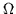=10). The strength of the quadrupole-quadrupole (QQ) interaction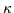provides here a suitable energy scale, and in the following all the energies and parameters are expressed as ratios with respect to.

Exact ground-state energies for all particle numbers N=0,1,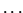,20 and G=0 are plotted in Fig. 9. All the even systems have the ground-state spins of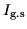=0, while in the odd systems, the ground-state spins are=|N-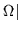/2+/2; this corresponds to rotational bands based on oblate sequences of deformed single-particle levels (see the discussion in Ref. ).

Even in a large energy scale of Fig. 9, the effect of the twofold Kramers degeneracy and the OES effect are clearly seen. Note that in this model the pure QQ interaction generates a (weak) OES effect. Indeed, in a relatively small phase space, the QQ interaction has a tangible pairing component.

In order to quantify the OES and mean-field effects, Fig. 10 shows values of the three-mass filter (1) obtained for different pairing strengths G. Ground-state energies of even and odd systems are used to calculate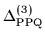for each value of G. For even systems, the ground-state spins equal 0 for all values of N and G, while for odd systems, the values of=19/2 (for all N) replace at large G the values of=|N-/2+/2, which characterize the G=0 solutions. This corresponds to a gradual transition from deformed to spherical shapes and from rotational to seniority-like excitation spectra.

In Fig. 10, one can clearly see that the OES increases almost linearly with G, while the pattern of alternating larger and smaller values ofis almost independent of G. Both these features ofare explicated by using filters (6) and (8), which give values of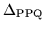and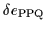plotted in Fig. 11. One can very well see the almost linear dependence of the OES on the pairing strength G, Fig. 11(a), and a very weak G-dependence (apart from N=10) of the single-particle energy spacings, Fig. 11(b).

The PPQ model exhibits several features pertaining to two kinds of phase transitions. First, the static pairing correlations set in at critical values of the pairing strength G. Depending on the number of particles, this phase transition occurs at about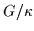=0.03-0.05 for even particle number. Second, the transition from deformed to spherical shapes occurs at slightly higher values of G, i.e., at about=0.08, 0.10, 0.12, and 0.12 for N=4, 6, 8, and 10, respectively. In addition, deformed equilibrium shapes are oblate for N=2, 4, 6, and 8, and prolate for N=10. (Note that apart from a linear dependence of energies on N, the PPQ Hamiltonian is exactly symmetric with respect to the particle-hole transformation N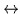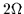-N .)

Since the system is finite (and fairly small for that matter), the phase transitions are hardly visible in the exact results of Fig. 11. However, when filters (6) and (8) are applied to the mean-field (HFB) ground-state energies (Fig. 12), the phase transitions become visible as sudden increases in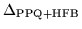(pairing transition), and degeneracies of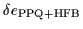(shape transition).

As one can see, a comparison of the exact and HFB ground-state energies (Figs. 11 and 12) is very instructive when it is based on comparing the corresponding filters (6) and (8). It turns out that in the PPQ model the HFB method reproduces quite well the OES and the single-particle properties simultaneously. Some deviations occur only near the phase transitions, where it is well known that the mean-field approximation is not accurate.

When analyzing exact solutions for systems interacting with two-body interactions, or when similarly analyzing the experimental data, one does not have a priori access to the single-particle energies or to the single-particle energy-spacings. In fact, the single-particle energies are concepts that appear naturally only in the mean-field approximation. Therefore, in order to assess the meaning of numbers obtained from the energy-spacing filter (8), one should compare them with the energy-spacings calculated directly from the mean-field spectra, i.e., with the differences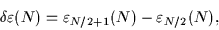(65)

[cf. Eq. (9)] where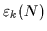is the kth (twofold degenerate) single-particle energy obtained within the mean-field approximation. Since a consistent application of the mean-field approximation to an odd system requires the time-reversal breaking, the Kramers degeneracy is lifted in odd systems. Consequently, in Eq. (65) one should only use the single-particle energies obtained self-consistently for systems with an even number of particles.

In Fig. 13(b) we show the differences (65) calculated from the single-particle spectra of canonical HFB energies obtained in the PPQ model. (In fact, since all of the PPQ+HFB equilibrium solutions conserve the axial symmetry, the PPQ+HFB method reduces to the simple BCS approximation, and the canonical energies are equal to the eigenenergies of the mean-field Hamiltonian.) It is clear that the energy-spacing filters (applied either to the exact or to the HFB total energies) give results similar to the differences of canonical energies only for deformed shapes. Whenever the mean-field solutions become spherical, differences (65) collapse to zero, as expected, while the energy-spacing filters give non-zero values. This result is easy to understand: in the spherical limit, the results of the PPQ model should resemble those of the seniority model. While the single-particle energies are degenerate (the Hilbert space consists of one j-shell only), the energy-spacing filter should be proportional to the pairing strength G (see Sec. 3.2). On the other hand, before the transition to sphericity, the results presented in Figs. 11(b), 12(b), and 13(b) are encouragingly similar. Note that deviations obtained at N=10 should again be attributed to the differences in predicted shapes (prolate vs. oblate).

Finally, in Fig. 13(a) we show the values of the HFB equivalent'' gap parameters (56) calculated in the PPQ+HFB model. It is seen that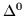significantly underestimates the magnitude of the OES effect, and moreover, it exhibits some particle-number dependence which is absent in the exact results.

In light of the above discussion, the very weak average dependence ofon N (except for the OES, of course), shown in Fig. 10, can be given by a very simple explanation. In the weak pairing limit,is small, and its overall particle-number dependence is much weaker than the even-odd effect (see Fig. 6). On the other hand, in the limit of strong pairing,is expected to approach the seniority limit in which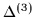depends only on the number parity but not on N. Note that the results of the non-degenerate model shown in Fig. 6 are very far from the spherical limit since G/d<1.Next: Conclusions Up: Theoretical models Previous: Second-order perturbation results
Jacek Dobaczewski
2000-03-09